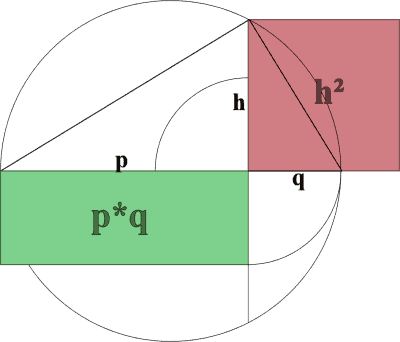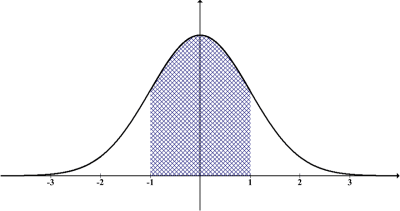# What Is Geometric Mean? (8 Common Questions Answered)

Geometric mean is less well-known than arithmetic mean (average).  However, geometric mean still has its uses, so it is helpful to know what it is, how to calculate it, and when to use it.

So, what is geometric mean?  The geometric mean of n numbers {x1, x2,…, xn} is the nth root of the product of the numbers, or n√(x1x2 … xn). We use all n of the xi values to calculate of the geometric mean. For n = 2 (x and y), the geometric mean is √(xy). For n = 3 (x, y, and z), the geometric mean is 3√(xyz).

Of course, geometric mean is always less than or equal to arithmetic mean.  Geometric mean is preferable to arithmetic mean in some contexts.

In this article, we’ll talk about geometric mean, what it is, how to calculate it, and when to use it.  We’ll also answer some common questions about geometric mean.

Let’s get started.

## What Is Geometric Mean?

Geometric mean is a type of mean (average) that takes the nth root of a product of n positive numbers.  In other words, to take the geometric mean of a set of numbers, we:

• multiply all of the values in the set together
• raise the product to the power of 1/n, where n is the number of values in the set

If a set of n numbers is A = {x1, x2, … , xn}, then the geometric mean formula is given by:

• n√(x1x2…xn)

or

• (x1x2…xn)1/n

We can see that for n = 2 numbers, the geometric mean is the square root of their product.  For a rectangle with side lengths x and y, the area would be xy, and the geometric mean √(xy) would give us the side length of a square with area xy.For two lengths p and q, the geometric mean h = √(pq) is the side length of a square with an area that is the same as the area of a rectangle with sides p and q.

Similarly, for n = 3 numbers, the geometric mean is the cube root of their product.  For a box with dimensions x, y, and z, the volume would be xyz, and the geometric mean 3√(xyz) would give us the side length of a cube with volume xyz.

### How To Calculate Geometric Mean

Let’s look at some examples with hard numbers so we can see how to calculate geometric mean.

#### Example 1: How To Calculate Geometric Mean Of Two Numbers

Let’s say we want to find the geometric mean of the numbers 2 and 18.

First, we find the product: 2*18 = 36.

Then, we take the square root of this product (since there are n = 2 numbers).

This gives us a geometric mean of √36 = 6.

So, the geometric mean of 2 and 18 is 6.

#### Example 2: How To Calculate Geometric Mean of Three Numbers

Let’s say we want to find the geometric mean of the numbers 5, 8, and 25.

First, we find the product: 2*8*25 = 1000.

Then, we take the cube root of this product (since there are n = 3 numbers).

This gives us a geometric mean of 3√1000 = 10.

So, the geometric mean of 5, 8, and 25 is 10.

#### Example 3: How To Calculate Geometric Mean of Four Numbers

Let’s say we want to find the geometric mean of the numbers 3, 4, 6, and 18.

First, we find the product: 3*4*6*18 = 1296.

Then, we take the fourth root of this product (since there are n = 4 numbers).

This gives us a geometric mean of 4√1296 = 6.

So, the geometric mean of 3, 4, 6, and 18 is 6.

### How To Calculate Geometric Mean In Excel

To calculate geometric mean in Excel, use the function GEOMEAN with an input of a set of numbers (or an array of numbers in consecutive cells).

For example, in Excel, we can find the geometric mean of 2 and 18 with the formula =GEOMEAN(2, 18).

Similarly, we can find the geometric mean of 5, 8, and 25 with the formula =GEOMEAN(5, 8, 25).

We can find the geometric mean of 12 numbers in cells A1 through A12 with the formula = GEOMEAN(A1:A12).  You can see this pictured below.

### Why Use Geometric Mean?

Geometric mean is used in some cases when arithmetic mean is not appropriate.  For example, if we wish to find the average compound growth rate (or rate of return) over multiple years, we would use geometric mean.

#### Example: Find The Compound Annual Growth Rate Of Business Revenue

Let’s say that a business has revenue that grows by 3% from year 1 to year 2, 8% from year 2 to year 3, and 4% from year 3 to year 4.We can use geometric mean to find compound annual growth rates for company revenue, profit, and share price over multiple years.

To find the compound annual growth rate, we take the geometric mean of the factors 1 + Rn (where Rn is the growth rate for the year n).

The factors in this case are 1.03, 1.08, and 1.04.  To find the geometric mean, we calculate:

• 3√(1.03)(1.08)(1.04)
• =3√(1.156896)
• =1.04978 (rounded)

Remember that 1.04978 is the growth factor, so we must subtract 1 to get the growth rate.  So, the compound annual growth rate of revenue for this business is 0.04978 (or 4.978% per year, on average).

### Can Geometric Mean Be Negative?

Geometric mean cannot be negative.  We always specify the positive real nth root (principal root) of the product of n numbers when we take a geometric mean.

Since all of the n numbers x1, x2, … , xn are positive, their product will be positive.  So, we will never have an even root of a negative number (which would lead to an imaginary number) or an odd root of a negative number (which would lead to a negative number).

### Does Geometric Mean Have Units?

Geometric mean does have units, and it has the same units as the original data values.  For example, if we have a set of n weights in pounds, then the geometric mean of those weights would be given in pounds.

This is similar to how units work for arithmetic mean (average) or median.

### Is Geometric Mean A Measure Of Central Tendency?

Geometric mean is a measure of central tendency, just like arithmetic mean (average) or median.  The geometric mean of a set of numbers will always be somewhere between the smallest and largest numbers in the set.

For example, we found earlier that:

• The geometric mean of 2 and 18 is 6, which is between 2 and 18.
• The geometric mean of 5, 8, and 25 is 10, which is between 5 and 25.
• The geometric mean of 3, 4, 6, and 18 is 6, which is between 3 and 18.

### Is Geometric Mean The Same As Median?

Geometric mean is not the same as median.  Although they are both measures of central tendency, they are calculated differently.

Remember that:

• Median is calculated by ordering the values in the data set from smallest to largest and then taking the middle data point.  If the data set has an even number of data points, then we take mean of the two middle data points to get our median.
• Geometric mean is calculated by taking the product of all the values in the data set and then taking the nth root of that product.

As you can see, we use every single value in the data set to calculate geometric mean, while we only use one or two values (the middle one or two numbers) to calculate the median.

If we increase the largest value (or decrease the smallest value) in a data set with 3 or more numbers, it will change the geometric mean, but not the median.The median is the value where 50% of the data points are greater and 50% are less. For a normal distribution, the median is the same as the arithmetic mean (average)

### Is Geometric Mean Less Than Arithmetic Mean?

Geometric mean is always less (or equal to) than arithmetic mean.  For n = 2 (x1 and x2), the inequality would look like this:

• (Arithmetic Mean) >= (Geometric Mean)

We can prove this as follows:

• (x1 – x2)2 > = 0  [since any real number squared is positive or zero]
• (x1 – x2)(x1 – x2) >= 0
• x12 – 2x1x2 + x22 >= 0  [use FOIL and combine like terms]
• x12 + 2x1x2 + x22 >= 4x1x2  [add 4x1x2 to both sides]
• (x1 + x2)2 >= 4x1x2  [factor left side as a perfect square trinomial]
• √(x1 + x2)2 >= √(4x1x2)  [take the square root of both sides]
• x1 + x2 >= 2√(x1x2)
• (x1 + x2) / 2 >= √(x1x2)  [divide by 2 on both sides]
• (Arithmetic Mean) >= (Geometric Mean)

To prove this for higher values of n (n = 3 and greater), we can use proof by mathematical induction or other methods.  You can find more detail on the general proof here.

## Conclusion

Now you know what geometric mean is and when you might use it.  You also know the answers to some common questions about this less common type of mean.

You might also want to read my article on arithmetic mean (average) and what it is used for.